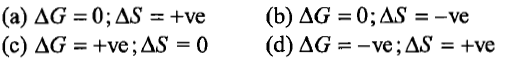(a) pressure is maintained constant

(b) gas is isothermally expanded

(c) there is perfect heat insulation

(d) the system changes heat with surroundings

Concept Questions :-

Internal Energy and work done
High Yielding Test Series + Question Bank - NEET 2020

Difficulty Level:

Heat of combustion $∆\mathrm{H}°$ for C(s), H2(g) and CH4(g) are -94, -68 and -213 kcal/mol. Then, $∆\mathrm{H}°$ for

C(s) + 2H2(g) $\to$CH4(g) is

(a) -17 kcal/mol                          (b) -111 kcal/mol

(c) -170 kcal/mol                        (d) -85 kcal/mol

Concept Questions :-

Thermochemistry
High Yielding Test Series + Question Bank - NEET 2020

Difficulty Level:

If 50 calorie are added to a system and system does work of 30 calorie on surroundings, the change in internal energy of system is:

(a) 20 cal                             (b) 50 cal

(c) 40 cal                             (d) 30 cal

Concept Questions :-

Enthalpy and It's Type
High Yielding Test Series + Question Bank - NEET 2020

Difficulty Level:

For the process:

H2O(l)[1 bar, 373 K] $\to$H2O(g)[1 bar, 373 K] the correct set of thermodynamic parameters are:Concept Questions :-

Spontaneity and Entropy
High Yielding Test Series + Question Bank - NEET 2020

Difficulty Level:

The internal energy change when a system goes from state A to B is 40 kJ/mol. If the system goes from A to B by a reversible path and returns to state A by an irreversible path. What would be the change in internal energy?

(a) 40 kJ                                    (b) >40 kJ

(c) <40 kJ                                  (d) Zero

Concept Questions :-

Internal Energy and work done
High Yielding Test Series + Question Bank - NEET 2020

Difficulty Level:

Change in entropy is negative for:

(a) Bromine (l)$\to$Bromine(g)

(b) C(s) + H2O(g) $\to$CO(g) + H2(g)

(c) N2(g,10 atm)$\to$N2(g,1 atm)

(d) Fe ( 1mol, 400 K) $\to$ Fe( 1mol, 300 K)

Concept Questions :-

Spontaneity and Entropy
High Yielding Test Series + Question Bank - NEET 2020

Difficulty Level:

The mathematical form of the first law of thermodynamics when heat (q) is supplied and W is work done by the system (+ve) is:

(a) $∆$U=q+W                        (b) $∆$U=q-W

(c) $∆$U=-q+W                       (d) $∆$U= -q-W

Concept Questions :-

Internal Energy and work done
High Yielding Test Series + Question Bank - NEET 2020

Difficulty Level:

Which statements are correct?

(a)  is called Clausius-Clapeyron equation

(b)  is called Trouton's rule

(c) Entropy is a measure of unavailable energy, i.e.,

unavailable energy = entropy x temperature

(d) All of the above

Concept Questions :-

Enthalpy and It's Type
High Yielding Test Series + Question Bank - NEET 2020

Difficulty Level:

$∆\mathrm{S}°$ will be highest for the reaction:

(a) Ca(s) + 1/2 O2(g) $\to$CaO(s)

(b) CaCO3(s)$\to$CaO(s) + CO2(g)

(c) C(s) + O2(g)$\to$CO2(g)

(d) N2(g) + O2(g)$\to$2NO(g)

Concept Questions :-

Spontaneity and Entropy
High Yielding Test Series + Question Bank - NEET 2020

Difficulty Level:

One mole of ice is converted into water at 273 K. The entropies of H2O(s) and H2O(l) are 38.20 and 60.01 J mol-1K-1 respectively. The enthalpy change for the conversion is:

(a) 59.54 J mol-1                                (b) 5954 J mol-1

(c) 595.4 J mol-1                              (d) 320.6 J mol-1

Concept Questions :-

Spontaneity and Entropy